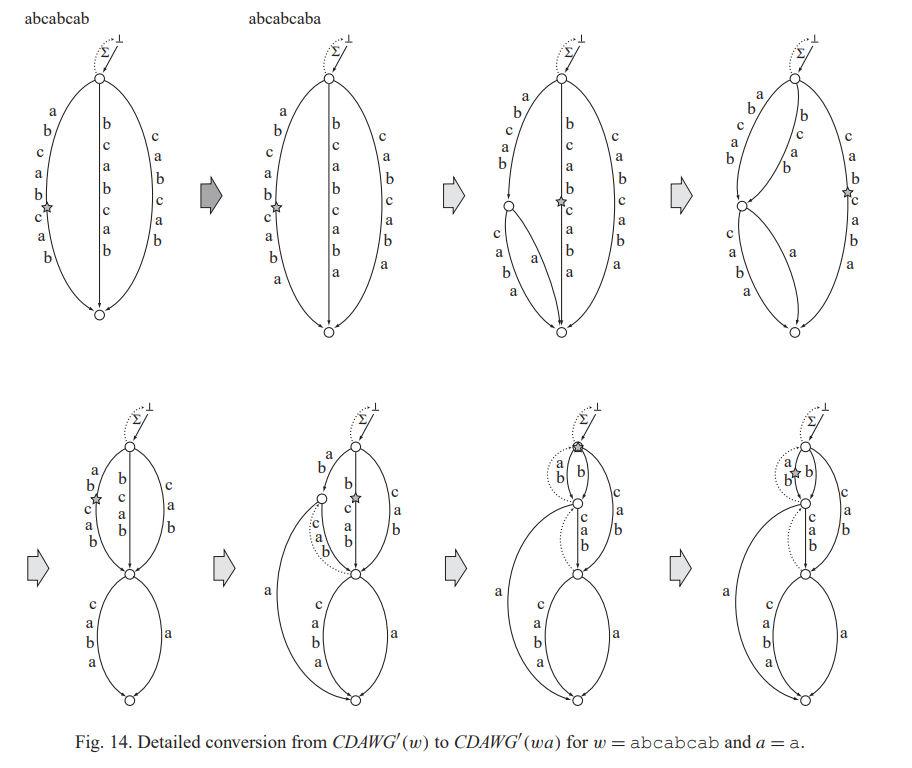# 从后缀尝试到在线线性紧致后缀金番茄

## 狂笑

Posted by ShanLunjiaJian's Blog on July 20, 2022

deepl有点拖拉机了，所以我用了生草机。然后就把au-tomaton翻译成了au番茄。

ukkonen算法所建立的后缀树定义为 :

• 每个点代表一个$$=_L$$等价类中的最长串。如果一个子串不对应一个点，或者说它不是等价类中的最长串，称它是一个隐式结点。否则它是一个显式结点。当我们说到点的时候，默认它是一个显示结点。为了记录一个隐式结点，我们可以使用三元组$$(u,c,p)$$，指从它的第一个显式父亲$$u$$出发，$$c$$开头的边上第$$p$$个位置对应的点。

• 每条边从一个点$$x$$连向另一个点$$xy$$，其中$$xy=\mathrm{RC}(xa)$$，$$a$$是一个字符；边上的串是$$y$$。这里$$y$$必然是原串的一个子串，所以我们用一个区间表示它。

• 考虑什么样的后缀不是一个叶子。显然一个点不是叶子，当且仅当有一个字符可以接到它后面，而后缀后面就是空了，所以它不是叶子当且仅当它在串中还有另一次出现(除了在末尾的那一次)。可以注意到长的后缀出现次数不会比短的多，所以我们找到最长的出现至少两次的后缀，称为 最长重后缀，设它的长度是$$l$$，那么所有长度$$\leq l$$的后缀都不是叶子，$$>l$$的都是叶子。

• 考虑 本来就有这么一条出边 的情况，可以注意到如果一个后缀有一条$$c$$开头的出边，那么比它短的后缀都有一条$$c$$开头的出边，所以也是长度$$\leq$$某个数的所有后缀是有的，剩下的都没有。ukkonen的一轮结束之后，$$cur$$会停在新的最长重后缀上，此时我们考虑它所在的等价类是否需要分裂。如果它是一个隐式结点，根据blumer那个按照$$\mathrm{maxlen}$$判断的方法，此时显然不需要分裂。如果它是一个显式结点，那么和blumer里面一样分裂即可。

sdptt2022 r2 D4 C. string

Sasha and Swag Strings

sdoi2022 子串统计

ptzsc18 Alice and Bob and A String